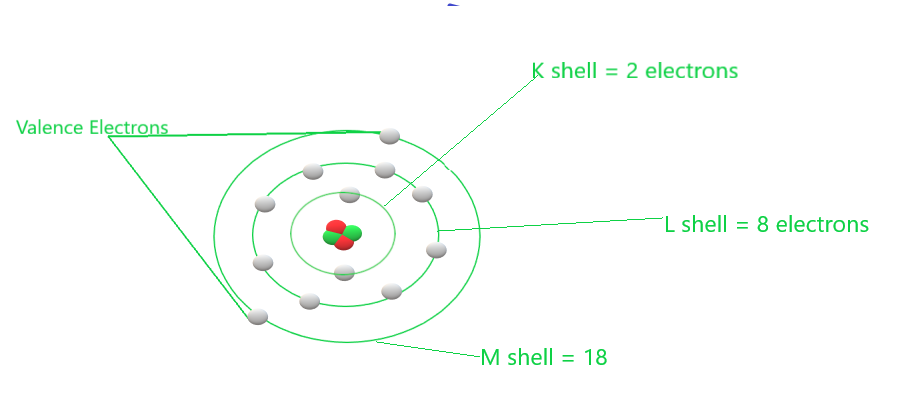How to Calculate Valency of Radicals?

• Last Updated : 18 Oct, 2021

To construct the formulas of ionic compounds, we must first understand the valencies of the positive and negative ions that combine to produce the ionic compounds. As a result, we’ll start with ion valencies. The valency of an ion is equal to its charge. If an ion has one unit charge, its valency is one, and it is referred to as a monovalent ion. When an ion contains two units of charge, its valency is two, and it is referred to as a divalent ion. And if an ion has three units of charge, its valency is three, and it is referred to as a trivalent ion. Please keep in mind that a cation has a positive charge and so has positive valency; an anion has a negative charge and thus has negative valency.

Radical behaves like a single atom with a positive or a negative charge on it, they are defined as an atom or a group of atoms of the same or different elements that shows the nature of the atom with chare on it. they have their own combining power due to their combining capacity they form chemical formulae.

For Example:

NH4+, Na+, NO3−, SO42−

The valency of any element is defined as a measure of its combining capacity and the number of electrons that must be gained or lost by an atom to obtain a stable electronic configuration.

Rules for writing of electrons configuration in different shells for the first 18 elements are formula to calculate the maximum number of electrons present in a shell = 2n2, where n = 1, 2, 3 (i.e. orbit number).

For different shells maximum electrons distributes as,

• K shell n = 1 = 2n2 =  2(1)2 = 2
• L shell n = 2  = 2n2 = 2(2)2 = 8
• M shell n = 3  = 2n2 = 2(3)2 = 18
• N shell n = 4  = 2n2 = 2(4)2 = 32Different shells are shown in the magnesium atom

The valency of charged ions or radicals is equal to the amount of charge present on them. On the basis of charge present on radicals or ions, they are divided into three:

1. Monovalent ions –  The ion having valency one or ions having one unit charge, are known as Monovalent ions. For example- Hydrogen ion (H+), lithium ion (Li+), ammonium ion (NH4+), Bromide ion (Br), Hydroxide ion (OH) etc.
2. Divalent ions – The ion having valency two or ions having two unit charge, are known as Divalent ions. For example- Magnesium ion (Mg2+), Calcium ion (Ca2+), Zinc ion (Zn2+), Oxide ion (O2-), sulphide ion (S2-) etc.
3. Trivalent ions – The ion having valency three or ions having three unit charge, are known as Trivalent ions. For example- Aluminum ion (Al3+), Iron ion (Fe3+), Nitride ion (N3−), Phosphate ion (PO43-) etc.

Valency of Common ions or radicals

Following is the tabular representation of common monoatomic cations:

Following is the tabular representation of common monoatomic anions:

Following is the tabular representation of common polyatomic radicals:

Sample Questions

Question 1: Find the number of atoms present in an H2S molecule and a PO43- ion.

In H2S molecule, there are two atoms of hydrogen (H) and one atom of sulphur (S). Hence three atoms of H and S totally present in H2S molecule. However, in PO43- ion, there are one atom of phosphorus (P )and four atoms of oxygen (O) hence five atoms of P and O totally present in PO43- ion.

Question 2: Calculate the molecular mass of each species given as,

H2O, Cl2, CO2 and CH4

The molecular masses of the given species are determined as shown below:

• The molecular mass of H2O =  2 × atomic mass of H + 1 × atomic mass of oxygen = 2 × 1  + 1 × 16  = 2 + 16 = 18 u.
• The molecular mass of Cl2 =  2 × atoms atomic mass of Cl = 2 × 35.5 = 71 u.
• The molecular mass of CO2 = 1 × atoms atomic mass of C + 2 × atomic mass of O = 1 × 12 + 2 × 16  = 12 + 32 = 44 u.
• The molecular mass of CH4 = 1 × atoms atomic mass of C + 4 × atomic mass of H = 1 × 12 + 4 × 1 = 16 u.

Question 3: What are polyatomic ions? Give examples.

Polyatomic ions are the ions that contain more than one atom but they are as a single unit. Examples of polyatomic ions are : CO32-, H2PO4–.

Question 4: Write the formulae of Sodium oxide and Aluminum chloride.

The formula of Sodium oxide can be calculated as,

Valency of Sodium = 1

Valency of Oxygen  = 2

Hence, the formula of sodium oxide is Na2O.

And the formula of Aluminum chloride can be calculated as,

Valency of Aluminum = 3

Valency of chloride = 1

Hence, the formula of Aluminum chloride is AlCl3.

Question 5: If 1 mole of carbon (C) atoms weighs 12 grams, what will be the mass of 1 atom of carbon?

Given that,

One mole of carbon (C) = 12 g weighs

one mole of carbon atoms = 6.022 × 1023

Molecular mass of carbon atoms = An atom of carbon mass = 12 g

Hence, mass of 1 carbon atom = 12 / 6.022 × 1023  = 1.99 ×10-23 g

My Personal Notes arrow_drop_up